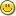### 1. DECIMALS

```I wrote a little function to round a number to a expected number
of decimals. File added. But I decouver that I cannot enter more
than 5 decimals in a atom. More decimals are automaticaly rounded
to 5 decimals. Are there any solution ? Thanks
J Hendrickx.
--RONDECI.EX -- round a number at a fixed number of decimals
include get.e
atom vv, i, dec
vv= 2.594567894  -- the number
dec=3           -- number of decimal

function rondxdec(atom n, integer dec) -- (number, nber of decimals to keep)
atom pivot, balance
pivot =0.5*(power(10,-dec))
balance=power(10,dec)
n=(floor((n + pivot) * balance))/balance
return n
end function

clear_screen()
i = rondxdec(vv,dec)
? i
```

### 2. Re: DECIMALS

```On Sun, 23 Feb 1997 16:10:45 +0100 Jean Hendrickx
<jean.hendrickx at INFOBOARD.BE> writes:

>I wrote a little function to round a number to a expected number
>of decimals. File added. But I decouver that I cannot enter more
>than 5 decimals in a atom. More decimals are automaticaly rounded
>to 5 decimals. Are there any solution ? Thanks
>J Hendrickx.
>--RONDECI.EX -- round a number at a fixed number of decimals
>include get.e
>atom vv, i, dec
>vv= 2.594567894  -- the number
>dec=3           -- number of decimal
>
>function rondxdec(atom n, integer dec) -- (number, nber of decimals to
>keep)
>atom pivot, balance
>pivot =0.5*(power(10,-dec))
>balance=power(10,dec)
>n=(floor((n + pivot) * balance))/balance
>    return n
>end function
>
>clear_screen()
>i = rondxdec(vv,dec)
>? i
>

Instead of using ? i OR print(1, i) use
printf(1, "%.16f\n", i)

You will notice that you have truly rounded past 5 decimals.
The print statement it designed to only display up to 5 decimal places.
but printf will print up to 16 decimal places.

ONE trick to it though

atom a, b

a = 1234567890.1234567  -- 17 places just as
b = .12345678901234567  -- 17 places

printf(1, "%.16f\n", a) -- shows "1234567890.1234570000000000" AND
printf(1, "%.16f\n", b) -- shows ".1234567890123457

Enjoy.--Lucius Lamar Hilley III
--   E-mail at luciuslhilleyiii at juno.com
--  I can only support file transfers of less than 64K and in UUE format.
```

### 3. Re: DECIMALS

```<luciuslhilleyiii at juno.com> wrotes
<Instead of using ? OR print(1,i) use printf(1,"%.16<n",i)
Thanks for thr trick, panic is over !Jean Hendrickx
```